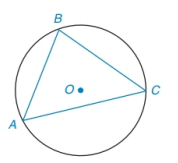Chapter 6.4, Problem 29E### Elementary Geometry for College St...

6th Edition
Daniel C. Alexander + 1 other
ISBN: 9781285195698

#### Solutions

Chapter
Section### Elementary Geometry for College St...

6th Edition
Daniel C. Alexander + 1 other
ISBN: 9781285195698
Textbook Problem
1 views

# Given that m ∠ A : m ∠ B : m ∠ C = 2 : 4 : 3 in circle O :a) Which angle is largest?b) Which chord is longest?(NOTE: See the figure for Exercise 26.)To determine

(a)

To find:

The largest angle.

Explanation

Given:

mA:mB:mC=2:4:3 in circle O. The figure given below,

Theorem Used:

In a circle (or in congruent circles) containing two unequal arcs, the larger arc corresponds to the larger central angle.

Calculation:

Since, mA:mB:mC=2:4:3 and the sum of the angles of a triangle is 180°, we get

mA+mB+mC=180°

Or 2x+4x+3x=180°

So

To determine

(b)

To find:

The longest chord.

### Still sussing out bartleby?

Check out a sample textbook solution.

See a sample solution

#### The Solution to Your Study Problems

Bartleby provides explanations to thousands of textbook problems written by our experts, many with advanced degrees!

Get Started